# 铜锁 SM2 算法性能优化实践（三）｜快速模逆元算法实现

08/23 14:31

## 优化方案比较选型

#### 1 拓展欧几里得算法求解模逆元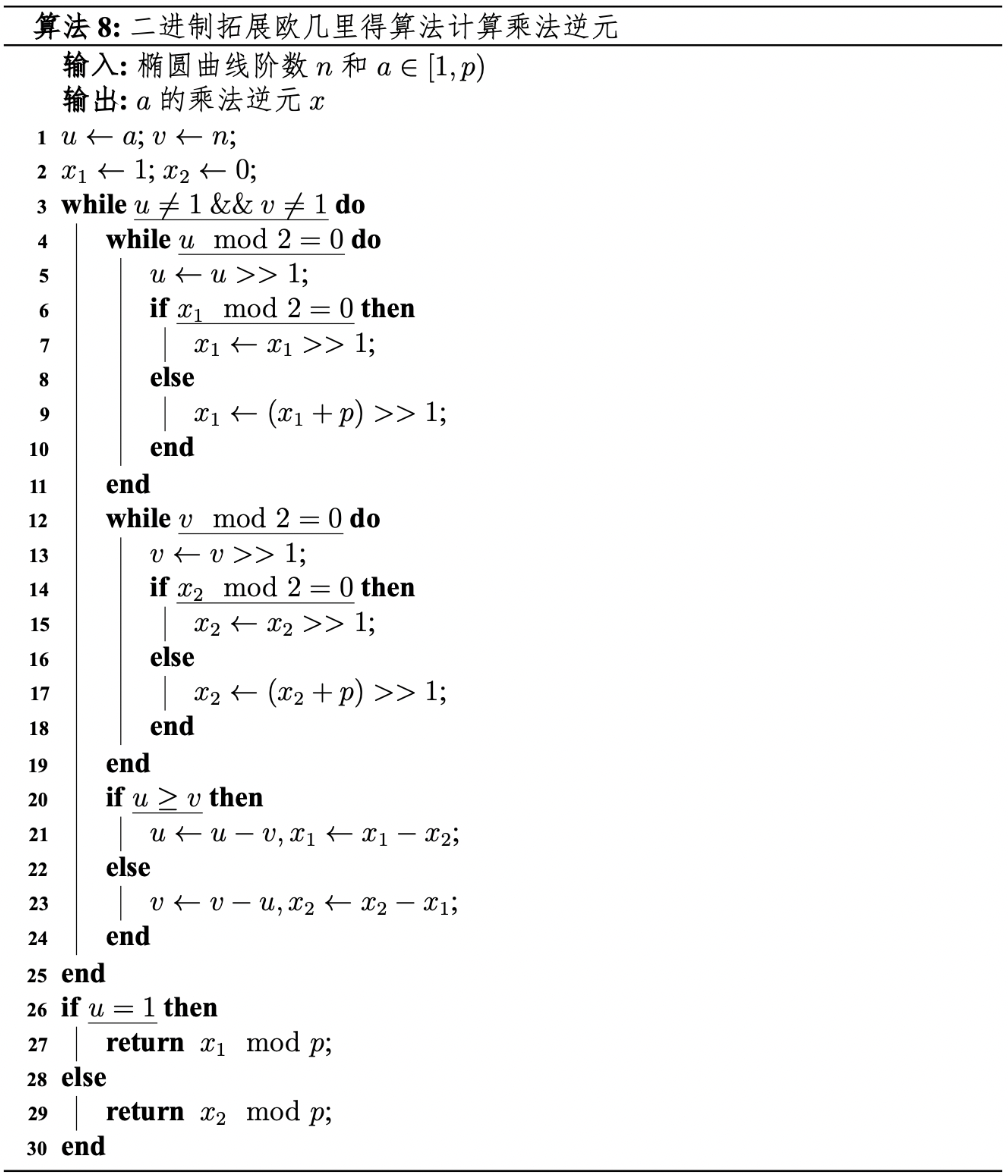#### 2 费马小定理求解模逆元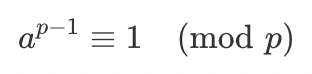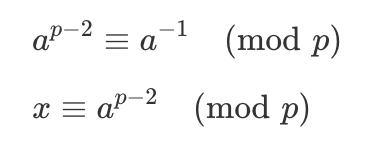## 算法实现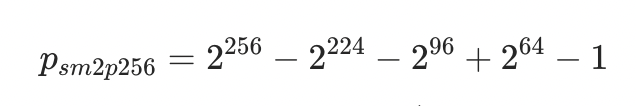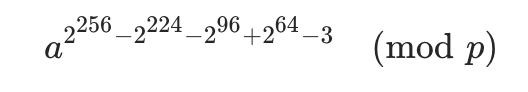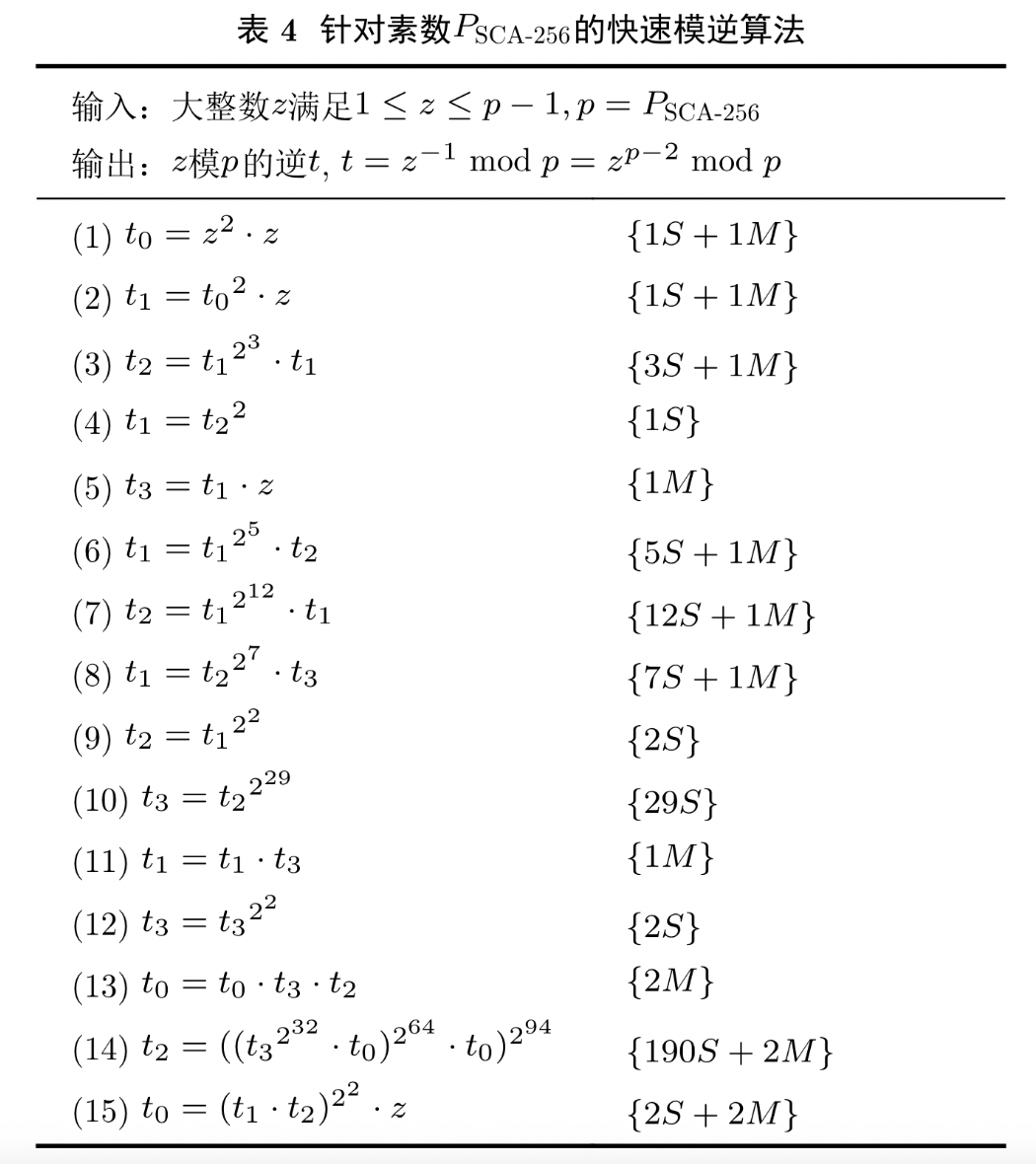static void felem_inv(felem out, const felem in)
{
felem t0, t1, t2, t3;
felem ftmp;
longfelem tmp;
unsigned i;

/* Step 1: t0 = a^3 = (2^2 - 2^0) * a */
felem_square(tmp, in);
felem_reduce(ftmp, tmp);
felem_mul(tmp, ftmp, in);
felem_reduce(t0, tmp);
/* Step 2: t1 = t0^2 * a = (2^3 - 2^0) * a */
felem_square(tmp, t0);
felem_reduce(ftmp, tmp);
felem_mul(tmp, ftmp, in);
felem_reduce(t1, tmp);

/* 部分中间代码略，完整代码可见/crypto/ec/sm2p256.c*/

/* Step 14: t2= ((t3^(2^32) * t0)^(2^64) * t0)^(2^94) = (2^254 - 2^222 - 2^94) * a */
felem_assign(ftmp, t3);
for (i = 0; i < 32; i++) {
felem_square(tmp, ftmp);
felem_reduce(ftmp, tmp);
}
felem_mul(tmp, ftmp, t0);
felem_reduce(ftmp, tmp);
for (i = 0; i < 64; i++) {
felem_square(tmp, ftmp);
felem_reduce(ftmp, tmp);
}
felem_mul(tmp, ftmp, t0);
felem_reduce(ftmp, tmp);
for (i = 0; i < 94; i++) {
felem_square(tmp, ftmp);
felem_reduce(ftmp, tmp);
}
felem_assign(t2, ftmp);
/* Step 15: out = (t1 * t2)^4 * a = (2^256 - 2^224 - 2^96 + 2^64 -1) * a */
felem_mul(tmp, t1, t2);
felem_reduce(ftmp, tmp);
felem_square(tmp, ftmp);
felem_reduce(ftmp, tmp);
felem_square(tmp, ftmp);
felem_reduce(ftmp, tmp);
felem_mul(tmp, ftmp, in);
felem_reduce(out, tmp);
}



## 总结与展望

【 文中链接 】

针对素数 PSCA-256 的快速模逆算法：https://jeit.ac.cn/cn/article/doi/10.11999/JEIT2110490 评论
0 收藏
0